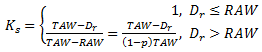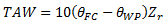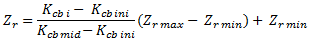# Potential and Actual Crop Evapotranspiration (ETc and ETa)

## Potential Crop Evapotranspiration (ETc)

The potential crop evapotranspiration under standard field conditions is calculated as follows:

ETc = ( Kcb + Ke ) ETref

## Actual Crop evapotranspiration (ETa)

Precipitation and irrigation amounts are often not sufficient to supply the full ETc requirement. In these situations, soil water content in the root zone is reduced to levels too low to permit plant roots to extract the full ETc amount. Under these conditions, water stress is said to occur, and ETa is less than ETc. The reduction in ETa can be estimated using a daily soil water balance, as follows. When field-specific estimates of ETa are needed, they can be estimated by:

ETa = Kact ETref

where

Kact = actual Kc value = Ks Kcb + Ke.

The stress coefficient, Ks is estimated as:where

Dr = root zone depletion, defined as the water shortage relative to field capacity [mm],
RAW = ‘readily’ available water [mm],
TAW = total available soil water in the root zone [mm],
p = depletion factor, the fraction of TAW that a crop can extract from the root zone without suffering water stress [0..1].

When Dr <= RAW, Ks = 1. At field capacity, Dr = 0. The degree of stress is presumed to progressively increase as Dr increases past RAW, the depth of readily available water in the root zone. The value for p varies by crop and crop growth stage, and typically ranges from about 0.4 for shallow-rooted crops to 0.6 for deep rooted crops. The Crop Library includes values for p ("depletion factor").

TAW is estimated as the difference between the water content at field capacity and wilting point (% vol):where

Zr = effective rooting depth [m]

For crop stage 1, Zr = Zr min  For crop stages 3 and 4, Zr = Zr max  For crop stage 2, Zr is estimated as:where

Zr min = minimum rooting depth for crop [m]
Zr max = maximum rooting depth for crop [m]

RAW is estimated as:

RAW = p TAW

The calculation of Ks requires a daily water balance computation for the root zone.